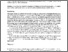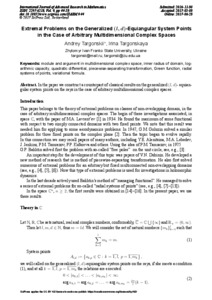# Extremal Problems on the Generalized (l, d)-Equiangular System Points in the Case of Arbitrary Multidimensional Complex Spaces

Targonskii, A. L. and Targonskaya, I. I. (2017) Extremal Problems on the Generalized (l, d)-Equiangular System Points in the Case of Arbitrary Multidimensional Complex Spaces. International Journal of Advanced Research in Mathematics (9). pp. 44-53. ISSN 2297-6213Preview
Text

## Abstract

In the paper we construct a counterpart of classical results on the generalized (l, d)- equiangular system points on the rays in the case of arbitrary multidimensional complex spaces.

Item Type: Article module and argument in multidimensional complex space, inner radius of domain, logarithmic capacity, quadratic differential, piecewise-separating transformation, Green function, radial systems of points, variational formula. Q Science > QA Mathematics > Mathematical Analysis Faculty of Physics and Mathematics > Department of Mathematical Analysis Ірина Ігорівна Таргонська 27 Feb 2019 07:53 27 Feb 2019 07:53 http://eprints.zu.edu.ua/id/eprint/28467

### Actions (login required)View Item